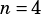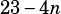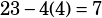Hey Mike, Is there a way do to this sequence problem without using the
“S=n[a1 + a…]” formula?
In an arithmetic sequence, each term after the first is found by adding the same number to the preceding term. If the sum of the first six terms of an arithmetic sequence is 78 and the sixth term is 23, what is the second term?
A) 3 B) 4 C) 7 D) 10 (The correct answer is 7)

Sure! (Although I don’t think you’d see this on the SAT—maybe a Subject Test.) You know that every term in the sequence is the same distance from its adjacent terms. Call that common difference n. Then you can say that the first six terms are:You know those  terms add to 78, so solve:If, then you can substitute into the expression we already have for the second term to get the answer. The second term is, sois the answer.